# Bihar Board 12th Physics Objective Answers Chapter 2 Electrostatic Potential and Capacitance

Bihar Board 12th Physics Objective Questions and Answers

## Bihar Board 12th Physics Objective Answers Chapter 2 Electrostatic Potential and Capacitance

Question 1.
Which of the following statement is not true ?
(a) Electrostatic force is a conservative force.
(b) Potential at a point is the work done per unit charge in bringing a charge from infinity to that point.
(c) Electrostatic force is non-conservative.
(d) Potential is the product of charge and work.
(b) Potential at a point is the work done per unit charge in bringing a charge from infinity to that point.Question 2.
I volt is equivalent to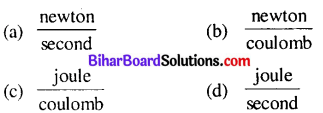$$\frac{\text { joule }}{\text { coulomb }}$$

Question 3.
The potential at a point due to a charge of 5 × 10-7 C located 10 cm away is
(a) 3.5 × 105 V
(b) 3.5 × 104 V
(c) 4.5 × 104 V
(d) 4.5 × 105 V
(c) 4.5 × 104 V
Solution:
(c) Here, q = 5 × 10-7C,r = 10cm = 0.1 m

Potential,Question 4.
A charge +q is placed at the origin O of x-y axes as shown in the figure. The work done in taking a charge Q from A to B along the straight line AB is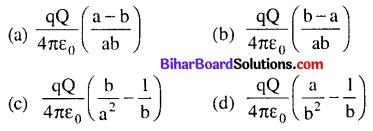(a) $$\frac{\mathrm{qQ}}{4 \pi \varepsilon_{0}}\left(\frac{\mathrm{a}-\mathrm{b}}{\mathrm{ab}}\right)$$Question 5.
The electric field and the potential of an electric dipole vary with distance r as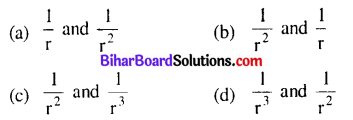(d) $$\frac{1}{r^{3}} \text { and } \frac{1}{r^{2}}$$

Question 6.
An electric dipole is placed at the centre of a hollow conducting sphere. Where of the following is correct ?
(a) Electric field is zero at every point of the sphere
(b) Electric field is not zero anywhere on the sphere
(c) The flux of electric field is not zero through the sphere
(d) All of these
(b) Electric field is not zero anywhere on the sphere

Question 7.
The distance between H+ and Cl ions in HCl molecules is 1.38 Å. The potential due to this dipole at a distance of 10 Å on the axis of dipole is
(a) 2.1 V
(b) 1.8 V
(c) 0.2 V
(d) 1.2 V
(c) 0.2 VQuestion 8.
A cube of side x has a charge q at each of its vertices. The potential due to this charge array at the centre of the cube is(b) $$\frac{4 q}{\sqrt{3} \pi \varepsilon_{0} x}$$

Question 9.
A hexagon of side 8 cm has a charge 4 µC at each of its vertices. The potential at the centre of the hexagon is
(a) 2.7 × 106 V
(b) 7.2 × 1011 V
(c) 2.5 × 1012 V
(d) 3.4 × 104 V
(a) 2.7 × 106 V
Solution:
As shown in the figure, O is the centre of hexagon ABCDEF of each side 8 cm. As it is a regular hexagon OAB, OBC, etc are equilateral triangles,

∴OA = OB = OC = OD = OE = OF = 8 cm = 8 × 10-2 m The potential at O isQuestion 10.
Equipotential surfaces
(a) are closer in regions of large electric fields compared to regions of lower electric fields
(b) will be more crowded near sharp edges of a conductor
(c) will always be equally spaced
(d) both (a) and (b) are correct
(d) both (a) and (b) are correct

Question 11.
What is the angle between electric field and equipotential surface ?
(a) 90° always
(b) 0° always
(c) 0°to90°
(d) 0° to 180°
(a) 90° alwaysQuestion 12.
The work done to move a unit charge along an equipotential surface from P to Q
(a) must be defined as $$-\int_{\mathrm{P}}^{Q} \overrightarrow{\mathrm{E}} \cdot \overrightarrow{\mathrm{d} \ell}$$
(b) is zero
(c) can have a non-zero value
(d) both (a) and (b) are correct
(d) both (a) and (b) are correct

Question 13.
The work done in carrying a charge q once round a circle of radius a with a charge Q at its centre is(d) zero

The formula for potential energy Calculator depends on the force acting on the two objects.

Question 14.
A test charge is moved from lower potential point to a higher potential point. The potential energy of test charge will
(a) remains the same
(b) increase
(c) decrease
(d) becomes zero
(c) decrease

Question 15.
Which among the following is an example of polar molecule ?
(a) O2
(b) H2
(c) N2
(d) HCl
(d) HClQuestion 16.
Dielectric constant for a metal is
(a) zero
(b) infinite
(c) 1
(d) 10
(b) infinite

Question 17.
Metallic sphere of radius R is charged to potential V. Then charge q is proportional to
(a) V
(b) R
(c) both V and R
(d) none of these
(c) both V and R

The buffer Capacity can be defined as the differential ratio of the increase in the amount of strong acid or strong base added, to pH variation.

Question 18.
In a parallel plate capacitor, the capacity increases if
(a) area of the plate is decreased
(b) distance between the plates increases
(c) area of the plate is increased
(d) dielectric constant decreases.
(c) area of the plate is increased

Question 19.
A parallel plate capacitor having area A and separated by distance d is filled by copper plate of thickness b. The new capacity is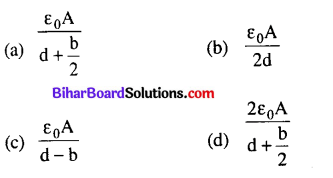(c) $$\frac{\varepsilon_{0} A}{d-b}$$

Question 20.
Figure shows the Held lines of a positive point charge. The work done by the field in moving a small positive charge from Q to P is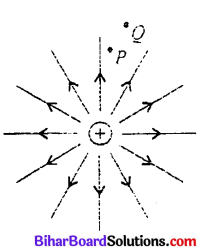(a) zero
(b) positive
(c) negative
(d) data insufficient
(c) negative

Question 21.
The charge on 3 μF capacitor shown in the figure is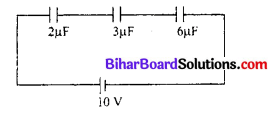(a) 2 μC
(b) 10 μC
(c) 6 μC
(d) 8 μC
(b) 10 μCQuestion 22.
Minimum number of capacitors each of 8 μF and 250 V used to make a composite capacitor of 16 μF and 1000 V are
(a) 8
(b) 32
(c) 16
(d) 24
(b) 32
Solution:
(b) Minimum number of capacitors in each row

= $$\frac{1000}{250}=4$$
Therefore, 4 capacitors connected in series.
If there are m such rows, then total capacity = m x 2 = 16
∴ m = 16/2 = 8
∴ minimum number of capacitors = 4 x 8 = 32

Question 23.
A parallel plate capacitor is made by placing n equally spaced plates connected alternatively. If the capacitance between any two adjacent plates is C then the resultant capacitance is
(a) nC
(b) C/n
(c) (n + 1)C
(d) (n – 1)C
(d) (n – 1)C

Question 24.
A parallel plate air capacitor has a capacitance C. When it is half filled with a dielectric of dielectric constant 5, the percentage increase in the capacitance will be
(a) 400%
(b) 66.6%
(c) 33.3%
(d) 200%
(b) 66.6%

Question 25.
A capacitor of capacitance 700 pF is charged by 100 V battery. The electrostatic energy stored by the capacitor is
(a) 2.5 × 10-8 J
(b) 3.5 × 10-6 J
(c) 2.5 × 10-4J
(d) 3.5 × 10-4J
(b) 3.5 × 10-6 J
Solution:
(b) Here, C = 700 pF = 700 × 10-12 F, V = 100 V

Energy storedQuestion 26.
A 16 pF capacitor is connected to 70 V supply. The amount of electric energy stored in the capacitor is
(a) 4.5 × 10-12 J
(b) 5.1 × 10-8 J
(c) 2.5 × 10-12 J
(d) 3.2 × 10-8 J
(b) 5.1 × 10-8 J

Question 27.
A metallic sphere of radius 18 cm has been given a charge of 5 × 10-6 C. The energy of the charged conductor is
(a) 0.2 J
(b) 0.6 J
(c) 1.2 J
(d) 2.4 J
(b) 0.6 J
Solution: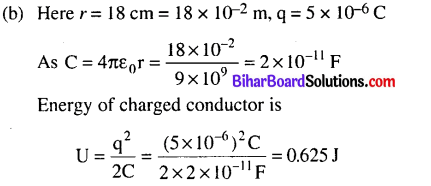Question 28.
Energy stored in a capacitor and dissipated during charging a capacitor bear a ratio
(a) 1 : 1
(b) 1 : 2
(c) 2 : 1
(d) 1 : 3
(c) 2 : 1
Solution:
(c) Half of the energy is dissipated during charging a capacitor.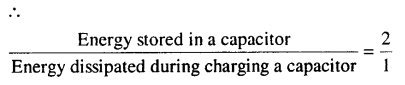Question 29.
Van deGraaff generator is used to
(a) store electrical energy
(b) build up high voltages of few million volts
(c) decelerate charged particle like electrons
(d) both (a) and (b) are correct
(b) build up high voltages of few million volts

Question 30.
Who established the fact of animal electricity ?
(a) Van deGraaff
(b) Count Alessandro Volta
(c) Gustav Robert Kirchhoff
(d) Hans Christian Oersted
(b) Count Alessandro VoltaQuestion 31.
In case of a Van de Graaff generator, the breakdown field of air is
(a) 2 × 108 V m-1
(b) 3 × 106 V m-1
(c) 2 × 1CT8 V m-1
(d) 3 × 104 V m-1
(b) 3 × 106 V m-1

Question 32.
A capacitor of 4 μF is connected as shown in the circuit. The internal resistance of the battery is 0.5 Ω . The amount of charge on the capacitor plates will be(a) 0
(b) 4 μC
(c) 16 μC
(d) 8 μC
(d) 8 μCQuestion 33.
Equipotentials at a great distance from a collection of charges whose total sum is not zero are approximately
(a) spheres
(b) planes
(c) paraboloids
(d) ellipsoids